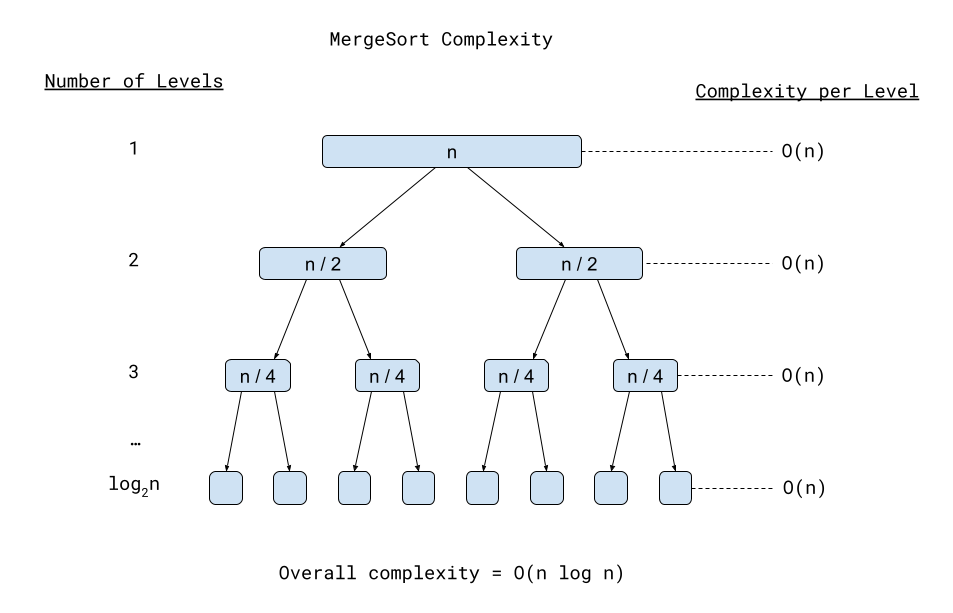# Merge Sort

example java arrays interviewing

Sorting an array is a Leetcode problem that presents this question:

Given an array of integers `nums`, sort the array in ascending order and return it.

You must solve the problem without using any built-in functions in `O(nlog(n))` time complexity

You can do this using an algorithm called merge sort.

Merge sort is one of the most famous recursive algorithms, and it sorts an array by recursively halving it into smaller and smaller subarrays, until the subarrays only have a single element in them. A single-element subarray is technically already sorted. It then merges the subarrays back together by selecting the smallest elements from each subarray in order, so that the result is sorted.

``````void mergeSort(int[] nums) {
mergeSort(nums, 0, nums.length - 1);
return nums;
}

// Recursive helper function that sorts the specified subarray.
// Note: startIndex and endIndex are inclusive boundaries.
void mergeSort(int[] array, int startIndex, int endIndex) {
// If the subarray is less than two elements, we're done.
if(startIndex >= endIndex) {
return;
}

// Split the subarray into two sub-subarrays and mergesort them.
int centerIndex = (startIndex + endIndex) / 2;
mergeSort(array, startIndex, centerIndex);
mergeSort(array, centerIndex + 1, endIndex);

// Merge the two sorted sub-subarrays into a single subarray.
merge(array, startIndex, centerIndex, endIndex);
}

// Merge two sorted halves of a subarray into a single sorted subarray.
// Note: startIndex and endIndex are inclusive boundaries.
void merge(int[] array, int startIndex, int centerIndex, int endIndex) {
// Create copies of the left and right halves.
// Arrays.copyOfRange endIndex is exclusive, so add one.
int[] leftArray = Arrays.copyOfRange(array, startIndex, centerIndex + 1);
int[] rightArray = Arrays.copyOfRange(array, centerIndex + 1, endIndex + 1);

int arrayIndex = startIndex;
int leftIndex = 0;
int rightIndex = 0;

// Look through the left and right halves, selecting the smallest from each in order.
while(leftIndex < leftArray.length && rightIndex < rightArray.length) {
if(leftArray[leftIndex] < rightArray[rightIndex]) {
array[arrayIndex] = leftArray[leftIndex];
leftIndex++;
} else {
array[arrayIndex] = rightArray[rightIndex];
rightIndex++;
}

arrayIndex++;
}

// Copy the remaining values from the left array into the output.
while(leftIndex < leftArray.length) {
array[arrayIndex] = leftArray[leftIndex];
leftIndex++;
arrayIndex++;
}

// Copy the remaining values from the right array into the output.
while(rightIndex < rightArray.length) {
array[arrayIndex] = rightArray[rightIndex];
rightIndex++;
arrayIndex++;
}
}
``````

# Algorithmic Complexity

The algorithmic complexity of recursive algorithms depend on exactly how you’re splitting up your data.

For merge sort, the algorithmic complexity is `O(n log n)`. Here’s an image that demonstrates why:Each “level” of the merge sort algorithm requires `O(n)` complexity, because it merges the sorted subarrays together. And since it splits the data in half each level, the number of levels will be `log2n`. So the total steps is `n * log2n`.

Similar to how you drop multipliers when converting from individual steps to big O notation, `n * log2n` becomes `O(n * log n)`.

That’s the algorithmic complexity for merge sort, but the algorithmic complexity of other recursive algorithms depends on what they’re doing. For example, the recursive `factorial()` function above has an algorithmic complexity of `O(n)`. I’ll talk more about recursive algorithmic complexity in the next tutorial.